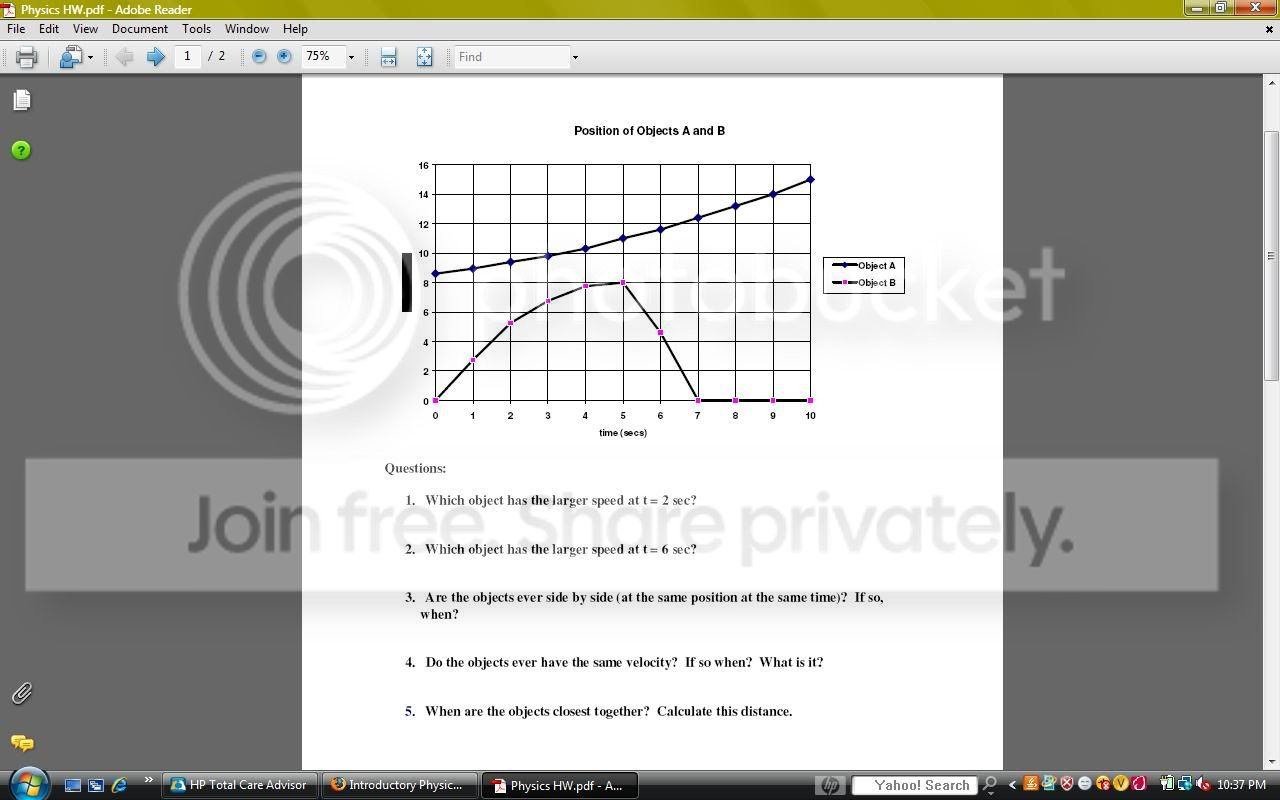# Physics Question

Physics Homework.For number one, it would be alright to calculate average speed because the slope of the line doesn't change, right?

I am lost on number two. I know it's asking for instantaneous speed and I have no idea how to find that. The book gives an example drawing a tangent line on a positive slope, but as you can see, object B is a negative slope, and I'm not even sure if that is the method I am supposed to perform anyway.

And because I am lost on number two, I am unable to complete the rest of the questions..

Thanks.

• Post a new comment